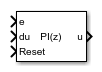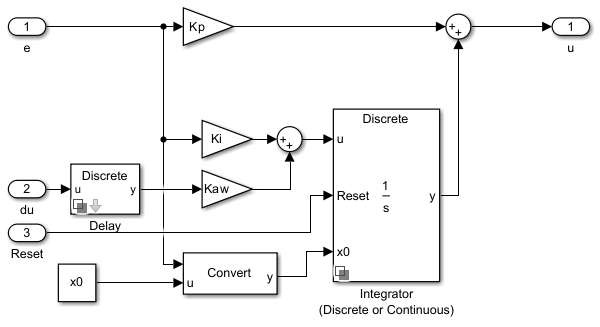# Discrete PI Controller

Discrete-time PI controller with external anti-windup input

• Library:
• Simscape / Electrical / Control / General Control

•## Description

The Discrete PI Controller block implements discrete PI control with external anti-windup input.

This diagram is the equivalent circuit for the controller with external anti-windup input.### Equations

The Discrete PI Controller block calculates the control signal using the backward Euler discretization method:

`$u\left(k\right)=\left[{K}_{p}+\left({K}_{i}+du\left(k\right){K}_{aw}\right)\frac{{T}_{s}z}{z-1}\right]\text{e}\left(\text{k}\right),$`

where

• u is the control signal.

• Kp is the proportional gain coefficient.

• Ki is the integral gain coefficient.

• Kaw is the anti-windup gain coefficient.

• Ts is the sampling period.

• e is the error signal.

To prevent excessive overshoot, the block can use back calculation to implement an external anti-windup mechanism. It inputs du(k), the difference between the saturated control signal, usat(k), and the calculated unsaturated control signal, u(k). It then multiplies the difference by the anti-windup coefficient and adds the amplified signal from the integral gain.

## Ports

### Input

expand all

Error signal, e(k), obtained as the difference between the reference, r(k), and measurement, y(k), signals.

Data Types: `single` | `double`

Difference, du(k), between the saturated u^sat(k) and the unsaturated control signals, u(k). If du(k) is zero, the anti-windup is disabled.

Description

Data Types: `single` | `double`

External reset (rising edge) signal for the integrator.

Data Types: `single` | `double`

### Output

expand all

Control signal, u(k).

Data Types: `single` | `double`

## Parameters

expand all

Proportional gain, Kp, of the PI controller.

Integral gain,Ki, of the PI controller.

Anti-windup gain, Kaw, of the PI controller.

Value of the integrator at simulation start time.

Time interval between samples. If the block is inside a triggered subsystem, inherit the sample time by setting this parameter to `-1`. If this block is in a continuous variable-step model, specify the sample time explicitly. For more information, see What Is Sample Time? and Specify Sample Time.

 Åström, K. and T. Hägglund. Advanced PID Control. Research Triangle Park, NC: ISA, 2005.